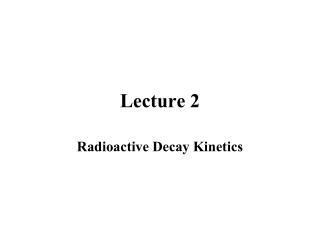DownloadDownload PresentationLecture 2

# Lecture 2

Télécharger la présentation## Lecture 2

- - - - - - - - - - - - - - - - - - - - - - - - - - - E N D - - - - - - - - - - - - - - - - - - - - - - - - - - -
##### Presentation Transcript

1. Lecture 2 Radioactive Decay Kinetics

2. Basic Decay Equations • Radioactive decay is a first order process, ie, the number of decays/s is proportional to the number of nuclei present • In eqn form, -dN/dt =λN where the constant λ is the decay constant • (A=λN) • Rearranging where N0 is the number of nuclei present at t=0.

3. If we remember the basic equation relating activity to number of nuclei in a sample, A=λN, then we can write Thus we have two equations that look the same, but have very different meanings Graphically

4. Decay constants, t1/2 Note that if t1/2 has units of time, λ has units of time-1 λ is probability per unit time of getting a decay

5. Use of basic decay equation What do these equations really mean? An easy decay rate to measure is 10 dpm of a nuclide with a t1/2 of 20 min. Then

6. Mean Life

7. Significance of mean life

8. Units 1 Bq=1 Becqueral=1 d/s 1Curie=1Ci=3.7x1010 Bq

9. Mixture of independently decaying activities

10. Radioactive Decay Equilibria Consider 123 rate of change of 2=rate of production of 2 by decay of 1 - rate of decay of 2

11. Special Cases • No equilibrium, product is stable (2=0)

12. Special Cases • Transient Equilibrium (λ2 ~ 10x λ1)

13. Note that the daughter activity is maximum at tmax

14. Special Cases • Secular Equilibrium (λ2 >> λ1)

15. Importance of Secular Equilibrium • Naturally occurring decay series

16. Importance of Secular Equilibrium • Production of radionuclides in a nuclear reaction • Nuclear reaction  2 

17. The “economics” of irradiating samples At t=∞, A=Asaturation

18. Bateman equations Consider the general case, 1 2  3  4...n Assume

19. Branching Decay Suppose a nucleus decays by several different modes, such as α-decay, SF-decay and EC decay. Then the total decay probability, λtot, is the sum of the probabilities of decaying by each mode, i.e.,λtot = λα+λSF+λEC Foe each mode of decay there is an associated partial halflife, i.e., t1/2(α)= ln 2 /λα

20. Naturally occurring radionuclides • Primordial • Cosmogenic • Anthropogenic

21. Environmentally interesting radionuclides • 222Rn • 40K • 3H

22. 14C

23. Simple Radionuclide dating Tricks • AMS • Variations in A0 or N0

24. Parent->Daughter Dating